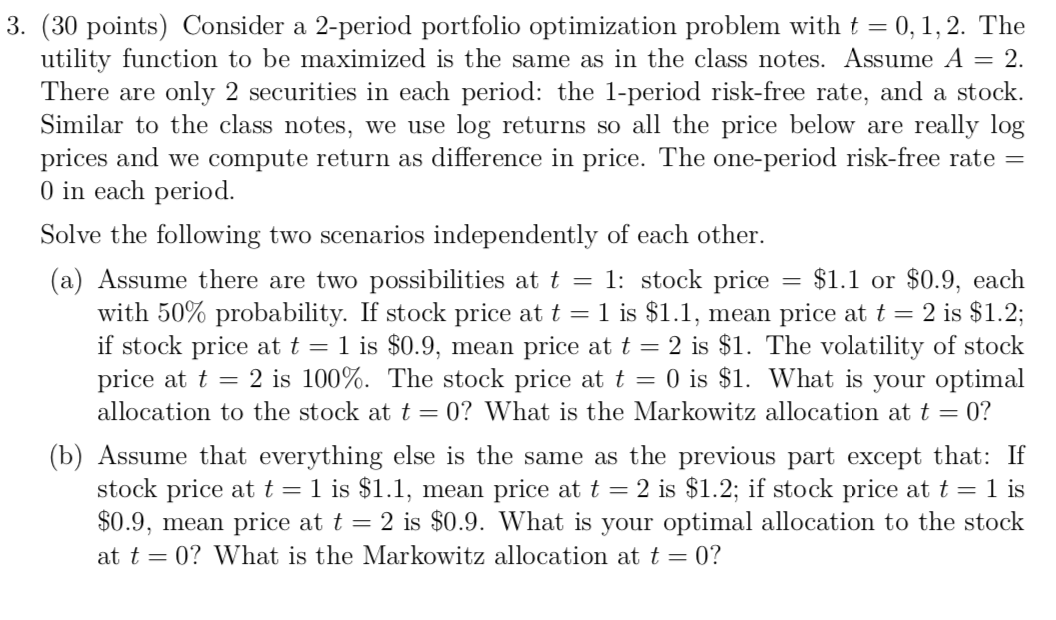### Create an Account

Home / Questions / Consider a 2-period portfolio optimization problem with t 01 2 The utility function

# Consider a 2-period portfolio optimization problem with t 01 2 The utility function3. (30 points) Consider a 2-period portfolio optimization problem with t = 0,1,2. The utility function to be maximized is the same as in the class notes. Assume A = 2. There are only 2 securities in each period: the l-period risk-free rate, and a stock. Similar to the class notes, we use log returns so all the price below are really log prices and we compute return as difference in price. The one-period risk-free rate = O in each period. Solve the following two scenarios independently of each other. (a) Assume there are two possibilities at t = 1: stock price = \$1.1 or \$0.9, each with 50% probability. If stock price at t = 1 is \$1.1, mean price at t = 2 is \$1.2; if stock price at t= 1 is \$0.9, mean price at t = 2 is \$1. The volatility of stock price at t = 2 is 100%. The stock price at t = 0) is \$1. What is your optimal allocation to the stock at t=0? What is the Markowitz allocation at t = 0? (b) Assume that everything else is the same as the previous part except that: If stock price at t = 1 is \$1.1, mean price at t = 2 is \$1.2; if stock price at t = 1 is \$0.9, mean price at t = 2 is \$0.9. What is your optimal allocation to the stock at t=0? What is the Markowitz allocation at t=0?

Feb 08 2020 View more View LessGet Solution